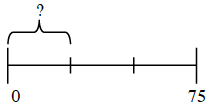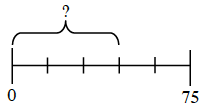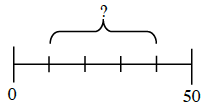### Home > CC1MN > Chapter 4 > Lesson 4.1.5 > Problem4-58

4-58.

What is the length of the marked portion of each line segment? Copy the segment onto your paper before finding the missing length. Assume that the entire line segment is subdivided into equal sections.

1.How many segments is $75$ broken into? Use this to figure out the value of each segment.

1.How many segments is $75$ broken into? Use this to figure out the value of each segment.

$45$. Be sure to show why this answer is correct.

1.How is this problem the same or different than parts (a) and (b)?